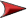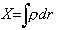# Interaction DepthNext# (g/cm2)The energy of charged particles is progressively absorbed by ionizing the matter it passes through. The more matter and the longer the distance, the more absorption. Cosmic rays pass through a tremendous variety of environments, from the almost absolute emptiness of extragalactic space to the relative clutter of our atmosphere, to the extreme density of our rocky Earth or even lead shielding. We need a measure of the path length that would help us predict the absorption. At any point along the path, the number of interactions is proportional to the density (r) times the path length (dr). If we were to add up all of these interactions along the particle's path, we would get a number that should be proportional to the total absorption.

If density has units of g/cm3 and the path length is in units of cm, then this Interaction Depth, X, has units of g/cm2. At first it seems pretty strange to be talking about some sort of distance with units of g/cm2! However, it does allow one to compare the effects of passage through miles of upper atmosphere, to passage through a few centimeters of water.

The idea of integrating along the particle's path is daunting. However, we already have some help in this. The pressure here at the surface of the earth, although partly due to dynamic effects of air movement, is mostly due to the total of all the weight of the air above that point. Pressure is force per unit area. Force is the total weight of all the air stacked above. Weight is mass times the acceleration due to gravity. Mass is density times volume. The cross-sectional area of a column of air radiating directly upward, gets larger as it rises. The acceleration of gravity decreases as you get farther away. However, the earth is so large and the atmosphere so thin, that both of these values are essentially constant (to within 1%).Thus at some altitude h, the pressure divided by g (=9.8m/ss) is a measure of the absorption along a vertical path to that point.

The 1967 Standard Atmosphere (see article later) gives us empirical equations to calculate the pressure at any altitude. The standard atmospheric pressure at sea level is defined as 101,325 Pa. The “depth” X, is therefore equal to ~10,000 kg/m2 or 1000 g/cm2. As divers know, a depth of 10 meters in water (density = 1 g/cm3) provides an additional atmosphere of pressure. In other words 10 meters of water will provide the same absorption as the entire thickness of the atmosphere.

 Material Density Thickness (g/cm3) 1 Atm. Equivalent Interstellar Space 10-23 100 million LY Air at 15,000 m (muon production zone) 0.00019 53,000 m Air at 12,500 m (max. KAO experiment) 0.00029 34,000 m Air at 4,000 m (Top of Mauna Kea) 0.00082 12,000 m Sea Level Air 0.00125 8,000 m Water 1 10 m Rock 5 2 m Iron 8 1.3 m Lead 11 0.9 m

 Altitude Note Density Pressure Depth ft m g/cm3 Pa g/cm2 233,000 71,000 Top of Std Atmosphere 6x10-8 67 0.7 105,000 32,000 Halfway 1x10-6 868 9 49,000 15,000 Zone of Muon production 2x10-4 12,000 130 41,000 12,500 Max. alt. KAO experiment 3x10-4 18,000 180 36,000 11,000 4x10-4 23,000 230 13,000 4,000 Top of Mauna Kea 8x10-4 62,000 630 0 0 Sea Level 1x10-3 101,000 1,000

## Slant Depth

All of the above Depth calculations are true only for muons arriving vertically. By simple trigonometry, it can be seen that dr' (distance along the slanted path) is equal to dr / cos (q). Where q is the angle of the path measured from vertical.

Given a Slant Depth, we can use the std atmosphere pressure equation to extrapolate an equivalent altitude that would correspond with this Depth if it were vertical. This will allow us, using ground level measurements, to extrapolate the Muon Intensity vs. Altitude graph from the KAO experiment to negative altitudes (i.e. below sea level).

In the following table: X' = X / cos (q), The Equivalent Altitude uses the 1st layer pressure equation from the Std Atmosphere model. The last three columns are provided as a comparison to the observed cos2 distribution to which the Particle Data book refers. The discrepancy is most likely due to muon decays. This might provide an interesting experiment of its own.

 q Slant Depth, X' (g/cm2) Equiv. Altitude (m) X0 /cos2 (q) (g/cm2) cos (q) cos2 (q) 0 ° 1,034 0 1,034 1 1 15 ° 1,070 -293 1,108 0.966 0.933 30 ° 1,194 -1230 1,378 0.866 0.750 45 ° 1,462 -3,022 2,068 0.707 0.500 60 ° 2,068 -6,249 4,135 0.500 0.250 75 ° 3,994 -13,000 15,432 0.259 0.067